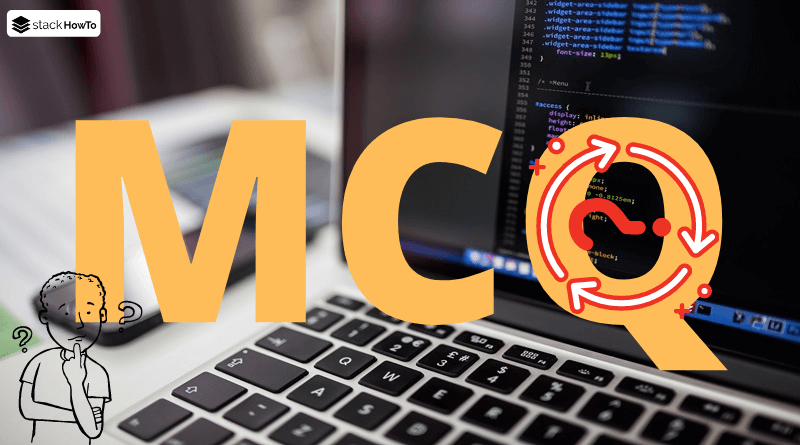MCQ

# PHP Questions and Answers – Functions – Part 2

This collection of PHP Multiple Choice Questions and Answers (MCQs): Quizzes & Practice Tests with Answer focuses on “PHP Functions”.

###### 1. Which the following declaration are valid function names?

A .function()

B function()

C \$function()

D €()

D
A valid function’s name can begin with a letter or underscore, followed-by any number of underscores, letters, or numbers. Based on the specified regular expression ([a-zA-Z_\x7f-\xff][a-zA-Z0-9_\x7f-\xff]*), a function’s name like this one is correct.

###### 2. Which PHP functions that begins with double underscore __________.

A Inbuilt Function

B Magic Function

C Default Function

D User Defined Function

B
PHP functions that begin with a double underscore are called magic functions in PHP.

###### 3. What is the result of the following PHP code?
```<?php
function a()
{
function b()
{
echo 'B';
}

echo 'A';
}

b();
a();
?>```

A BA

B AB

C BBA

D Error

D
Output:

```Fatal error:  Uncaught Error: Call to undefined function b() in [...][...]:12
Stack trace:
#0 {main}
thrown in [...][...] on line 12
```

###### 4. Which the following are the mathematical functions in PHP?

A pow

B exp

C log

D log5()

A, B, C
PHP offers many predefined math functions that can be used to achieve mathematical operations.

###### 5. Writing 2**4; will return the following result in PHP

A 2

B 4

C 8

D 16

D
The operator ** means exponentiation. 2 ** 4, Result of raising 2 to the 4’th power

###### 6. What is the result of the following PHP code?
```<?php
\$foo = "ipsum";

function fun(\$bar)
{
echo \$bar;
echo \$foo;
}

fun("lorem");
?>```

A loremipsum

B lorem

C ipsum

D Error

B
If you want to place some variables in function that was not passed by it, you should use “global”. Inside the function type `global \$foo`.

###### 7. What is the result of the following PHP code?
```<?php
function show(\$msg)
{
echo "\$msg";
}
\$display = "show";
\$display("Welcome To StackHowTo!");
?>```

A \$msg

B show

C Welcome To StackHowTo!

D Error

C
You can call a function using a variable which stores the function’s name.

###### 8. What is the result of the following PHP code?
```<?php
function prod(\$n1, \$n2, \$n3)
{
\$res = \$n1 * \$n2 * \$n3;

echo "The product is \$res";
}

prod(1, 2, 3, 4);
?>```

A The product is 24

B The product is 12

C The product is 6

D Error

C
The function accepts just three arguments, so it will not consider the last argument.

###### 9. Which function in PHP allow you to convert a string to an array?

A explode()

B to_array()

C implode()

D expand()

A
explode() function allow you to convert a string to an array.

###### 10. What is the result of the following PHP code?
```<?php
\$str = "HELLO";
\$str = lcfirst(\$str);
echo \$str;
?>```

A HELLO

B hello

C Hello

D hELLO

D
lcfirst() function allow you to convert the first character of a string to lowercase.MCQPractice competitive and technical Multiple Choice Questions and Answers (MCQs) with simple and logical explanations to prepare for tests and interviews.Read More